• 文章目录、基础概念插值是什么拟合是什么插值拟合的相同点插值拟合的不同点二、常用的基本插值方法高次多项式插值拉格朗日多项式插值牛顿插值差商矩阵低次多项式插值（不易震荡）分段线性插值Hermite插值三次...
文章目录摘要（必看）0 基础概念什么是插值插值用途什么是拟合插值和拟合的相同点插值和拟合的不同点1 常用的基本插值方法1.1 多项式插值法1.1.1 拉格朗日多项式插值法多项式插值并不是次数越大越好（龙格现象）分段低次线性插值以提高精度，减小插值误差分段二次插值分段线性插值拉格朗日插值的局限示例1.1.2 牛顿多项式插值法（差商）差商示例低次多项式插值（不易震荡）的其他示例1.1.3 拉格朗日插值和牛顿插值的对比不同相同1.2 考虑导数的插值方法1.2.1Hermite插值分段三次hermite插值1.2.2 三次样条插值示例用插值进行数据预测总结(必看+1)
摘要（必看）
本文特别长（长到需要些摘要·····），这对可读性造成很大负面影响，但我觉得都是插值，放在一起比较方便对比，所以目录逻辑做的比较清晰，实际上又臭又长的本文仅仅讲了两大类插值方法，一类是只考虑最基本插值条件的多项式插值，一类是还要考虑导数的插值，第一类又主要细分为拉格朗日多项式插值和牛顿多项式插值，且分别讲了基于他俩的分段低次插值，第二类主要细分为只考虑一阶导数相等的hermite插值和考虑一二阶导数相等的样条插值，并讲了基于他俩的三次分段插值。所有插值方法都给出了示例，并有图和代码。文末给出了精华总结。
另外，本文参考了很多清风老师的数学建模教程中有关插值的资料（部分公式、代码和文字描述），引用的文字部分都统一用灰框框起来的，清风老师的这套资料和视频讲解特别详细有用，可以在B站找到，虽然要花点小钱，但亲测真的非常值得。

文章太长，滚轮滚起来太慢，可以用crtl + home或者ctrl + end快速到达顶部和底部哦

0 基础概念
什么是插值
求出过已知个有限数据点的近似函数。

插值法是数值分析中最基本的方法之一。 在实际问题中遇到的函数是许许多多的，有的甚至给不出表达式，只供给了一些离散数据，例如，在查对数表时，需要查的数值在表中却找不到，所以只能先找到它相邻的数，再从旁边找出它的更正值，按一定的关系把相邻的数加以更正，从而找出要找的数，这种更正关系事实上就是一种插值。采用不同的插值函数，逼近的效果也不同。

当然啦，到后面你就会知道，已知数据点上取值相同只是插值的最基本条件，是必须满足的基础条件，这个都不满足就不是插值了。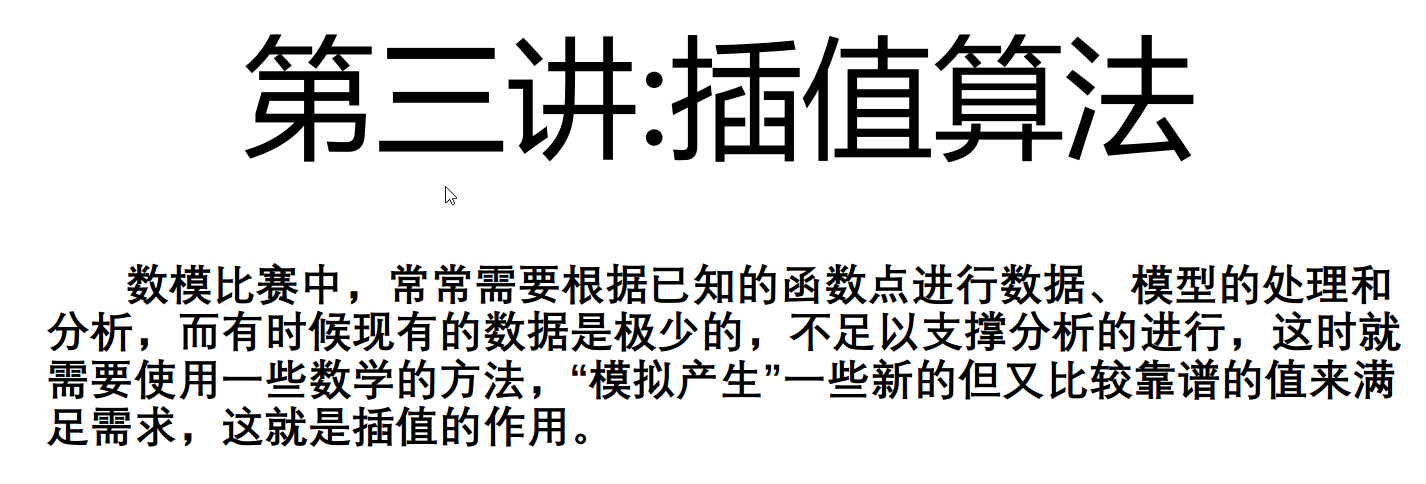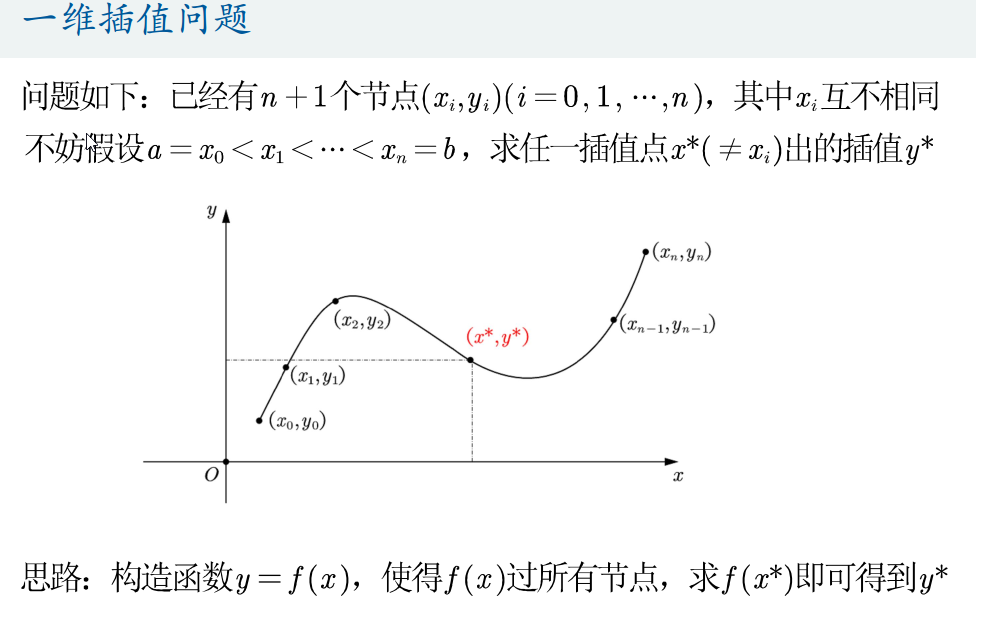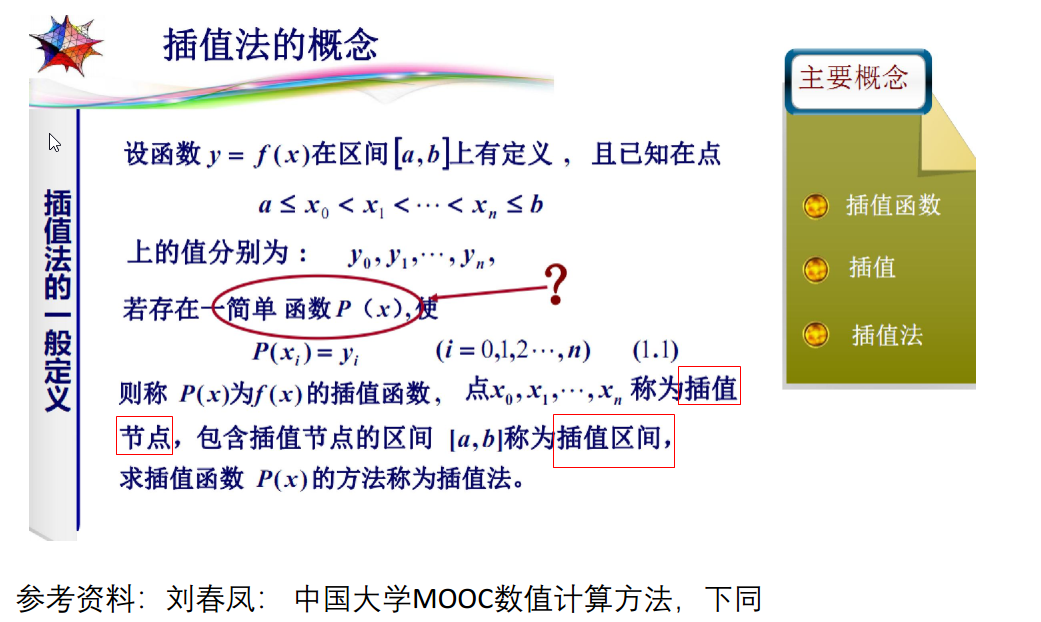插值用途
补全缺失数据
基于已知数据进行预测
什么是拟合
求出一个不要求过已知数据点的近似函数，不要求过那些数据点，只要求在这些点上的总偏差最小。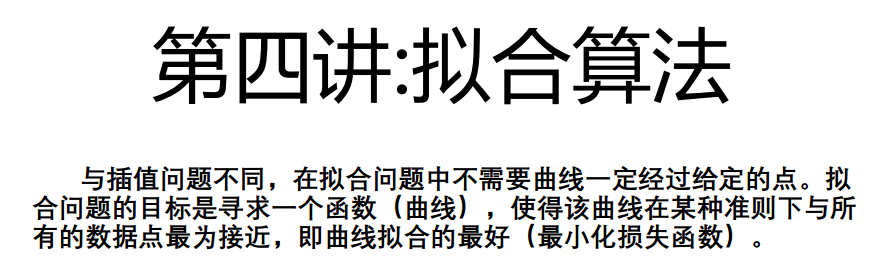插值和拟合的相同点
都是根据一组数据构造一个近似函数
插值和拟合的不同点
近似的要求不同；数学方法完全不同

一个问题到底应该插值还是拟合，有时容易确定有时不能明显看出。
我个人觉得数据点给的很多的时候就不再需要插值了，只需要拟合，看数据的走向趋势即可，因为本身插值就是在数据点太少的情况下看不出数据趋势才需要去生成新的可靠数据点的。另一方面是因为，数据点本身很多的话，多项式插值则次数很高，龙格现象会造成不准确插值。当然这一点可以通过分段低次插值克服，下面会讲

1 常用的基本插值方法
1.1 多项式插值法
不同的多项式插值方法都是去构造一个n次插值多项式

多项式插值是函数插值中最常用的一种形式。在一般的插值问题中，插值条件可以唯一地确定一个次数不超过 的插值多项式。从几何上可以解释为：可以从多项式曲线中找出一些不超过 次的点通过平面上 个不同的点。插值多项式有两种常用的表达式形式，一种是拉格朗日插值多项式，另一种是牛顿插值多项式，此外拉格朗日插值公式与牛顿插值公式永远相等。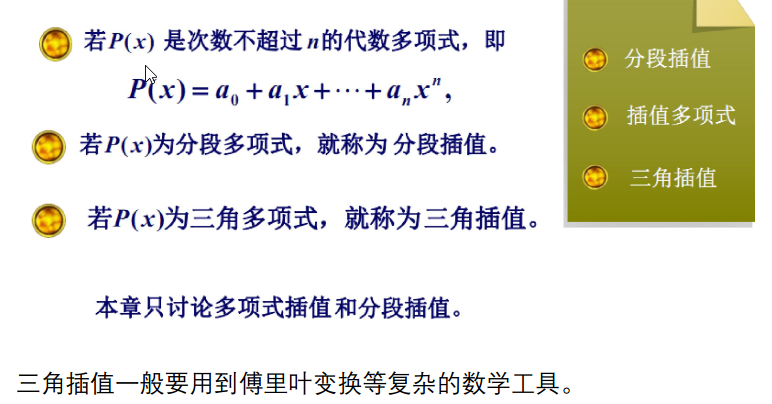只要n+1个节点互不相同，就一定存在唯一的n次多项式使得这n+1个点过这个多项式，厉害！！啊哈哈
若不限制多项式次数为n，就不唯一了哦。也是从证明里可以看出来的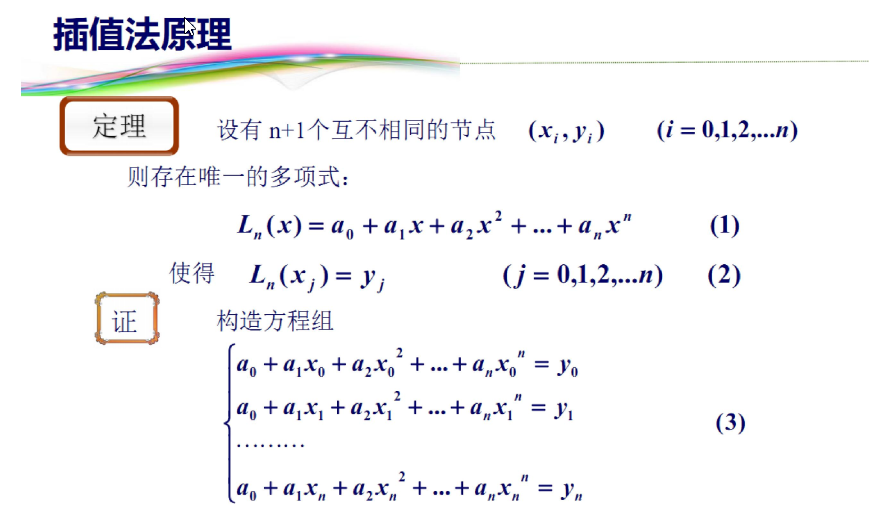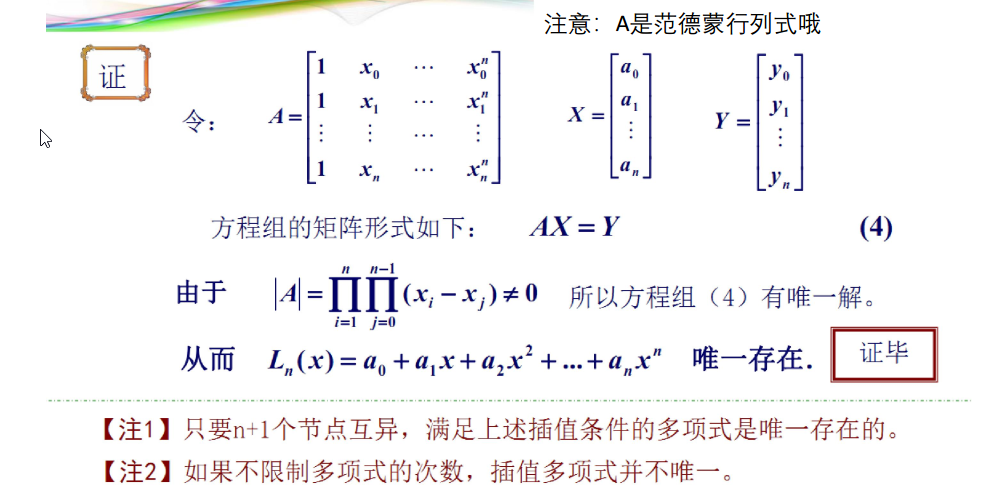1.1.1 拉格朗日多项式插值法
拉格朗日插值多项式是一种特殊的多项式形式，刚好过所有插值节点，是一种很凑巧的多项式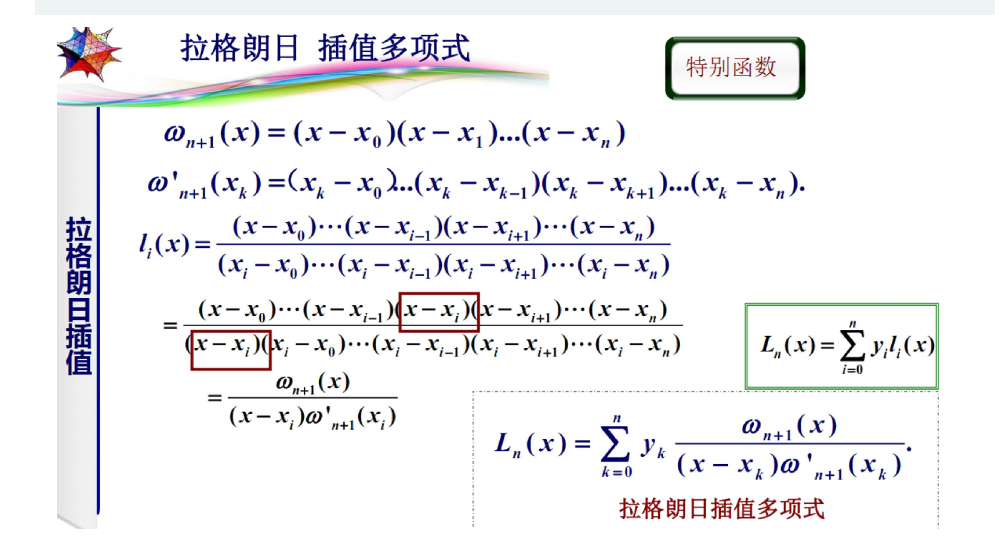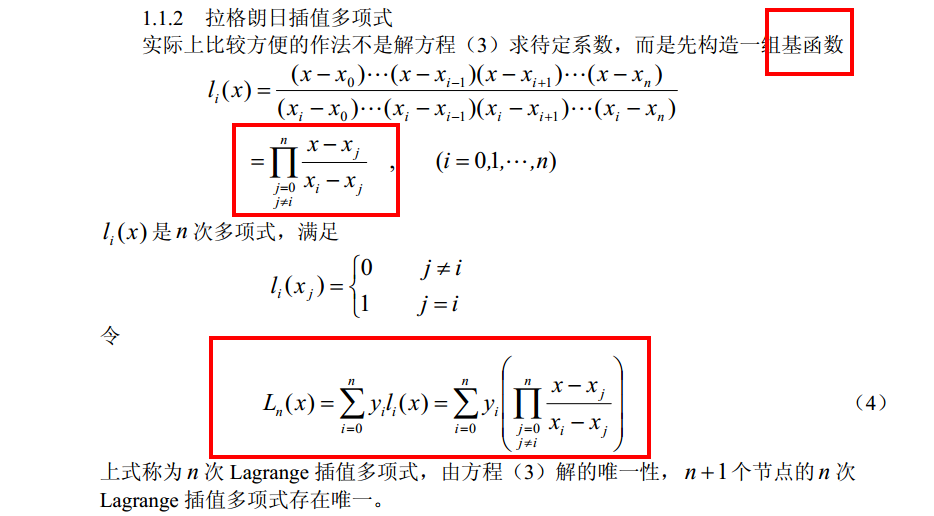例子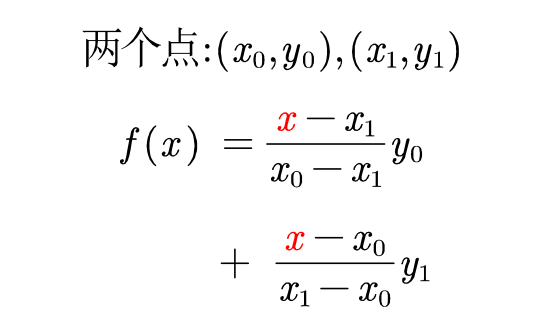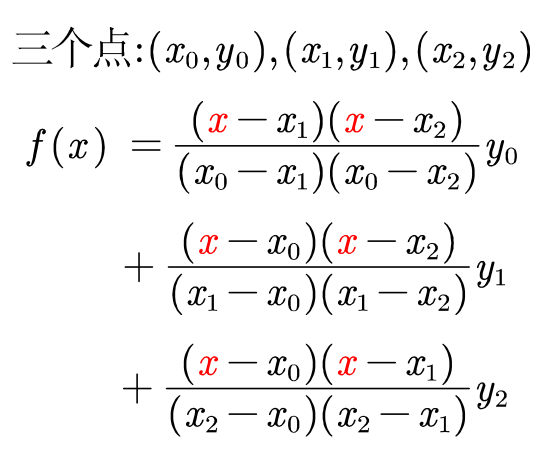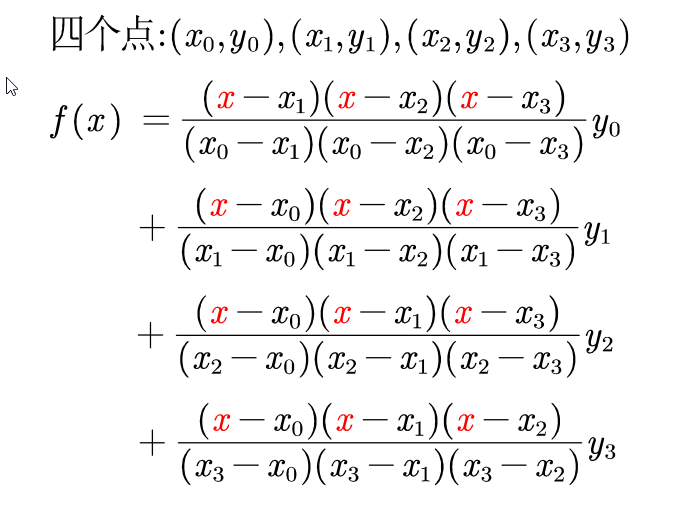所以拉格朗日多项式一共n项，n是已知数据点（插值节点）的数目
多项式插值并不是次数越大越好（龙格现象）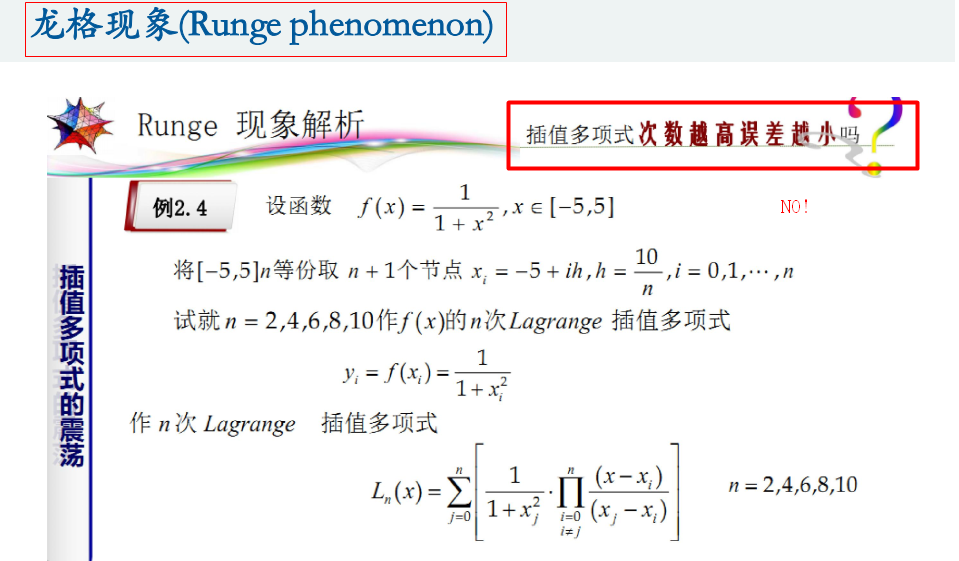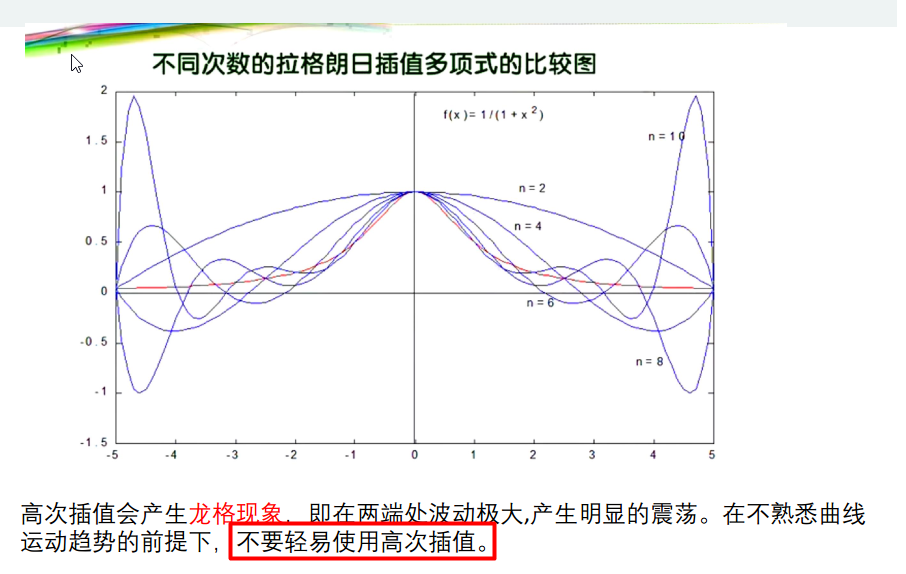分段低次线性插值以提高精度，减小插值误差
分段二次插值
只考虑最近的三个点，进行构造分段的拉格朗日插值多项式，以避免高次多项式插值带来的龙格效应，实现更高的精度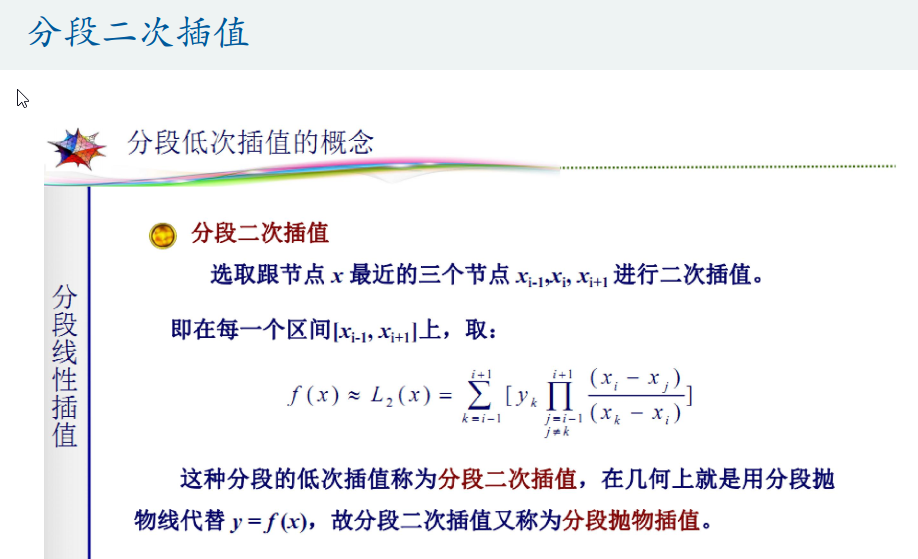这张图的公式有误，应该改为
$f(x)\approx L_2(x)=\sum_{k=i-1}^{i+1}[y_k \prod_{j=i-1 \atop j=\not k}^{i+1}\frac{(x-x_j)}{(x_k-x_j)}]$
实际就是分段线性插值
分段线性插值本质上是多项式插值，而且是加权式的插值方法。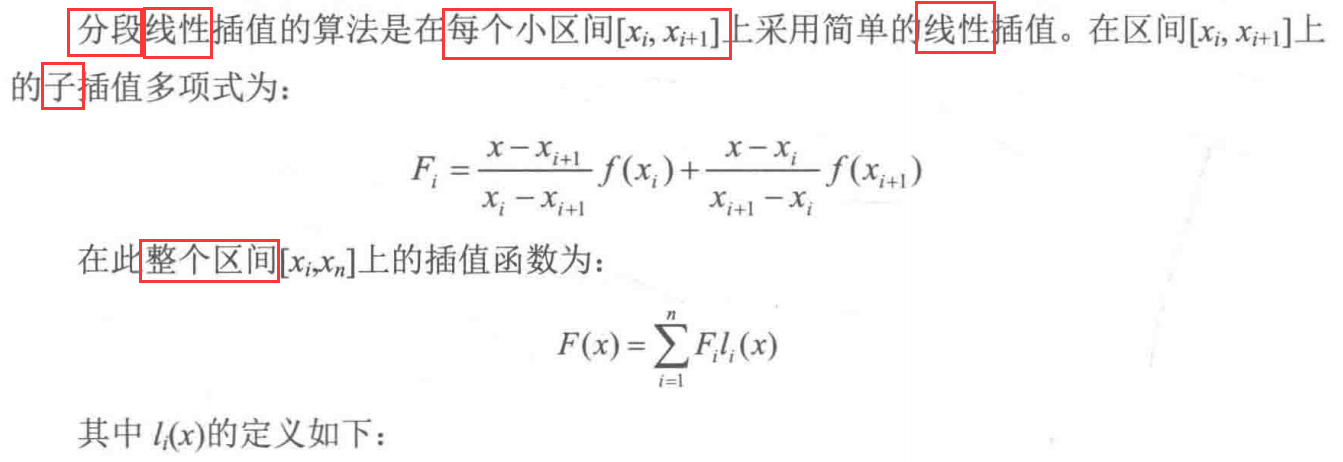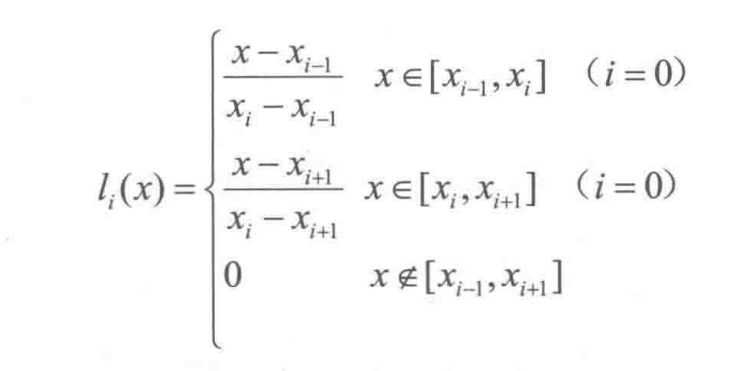第一个图中的$F_i$通分后：
$\frac{x_if(x_{i+1})-x_{i+1}f(x_i)}{x_i-x_{i+1}}+\frac{f(x_i)-f(x_{i+1})}{x_i-x_{i+1}}x$
这其实就是$(x_i,f(x_i))$和$(x_{i+1},f(x_{i+1}))$两点的连线的一次函数
所以分段线性插值的子插值函数没什么高深的，就是每段两个端点的连线。
$l_i(x)$就像是一个权重函数，我们用一个配巨丑手画图的例子来具体看看它为什么是权重
假设有5点已知数据点，$x_1,x_2,\cdots,x_5$,那么子插值函数$F_1,F_2,F_3,F_4$就分别是相邻两点的连线函数
现在对一个未知函数值的取值在$x_1,x_2$之间的点$x_c$进行插值，由于
l_1(x)=\left\{ \begin{aligned} &\frac{x-x_0}{x_1-x_0}&,&x \in[x_0,x_1]\\ &\frac{x-x_2}{x_1-x_2}&,&x \in[x_1,x_2]\\ &0&,& x \notin [x_0,x_2] \end{aligned} \right.\quad l_2(x)=\left\{ \begin{aligned} &\frac{x-x_1}{x_2-x_1}&,&x \in[x_1,x_2]\\ &\frac{x-x_3}{x_2-x_3}&,&x \in[x_2,x_3]\\ &0&,& x \notin [x_1,x_3] \end{aligned} \right.
所以$F(x_c)=F_1(x_c)\frac{x_c-x_2}{x_1-x_2}+F_2(x_c)\frac{x_c-x_1}{x_2-x_1}$
其中$\frac{x_c-x_2}{x_1-x_2}和\frac{x_c-x_1}{x_2-x_1}$分别是$x_c$到$x_2$的距离在$x_1,x_2$之间距离的比例，$x_c$到$x_1$的距离在$x_1,x_2$之间距离的比例
而$F_1(x_c)$则是$x_1,x_2$连线函数上$x_c$对应函数值，即下图图示中的a
$F_2(x_c)$则是$x_2,x_3$连线函数上$x_c$对应函数值，即下图图示中的b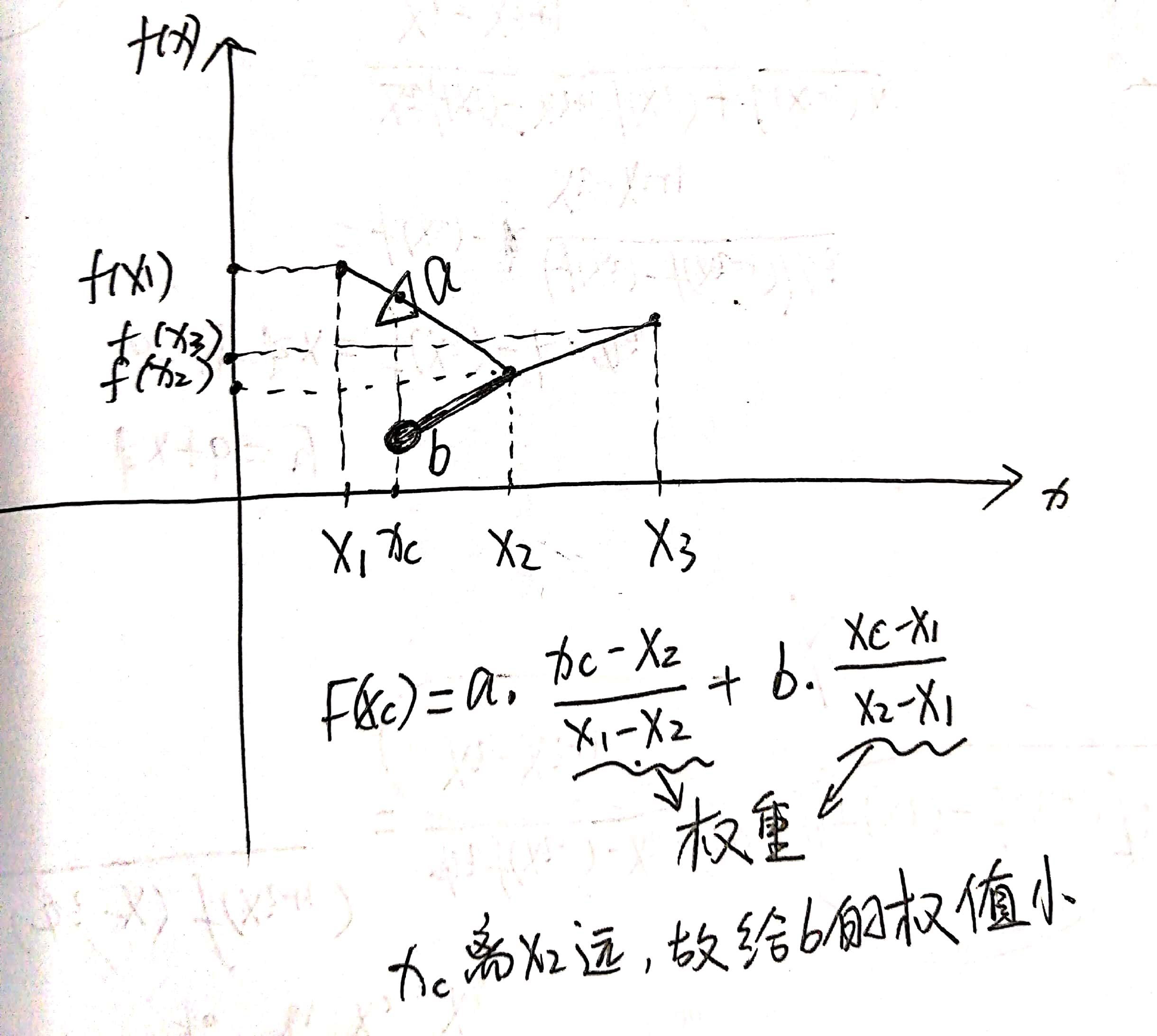n越大，分段越多，插值越好，误差越小
缺点：分段线性插值不能保证在节点处的插值函数的导数的连续性，即不光滑（看后面的示例）
拉格朗日插值的局限
从定义式可看到，每个基函数$l_i(x)$都需要利用所有插值节点来计算，所以一旦新增一个数据点，所有的基函数全部需要重新计算，所以计算量上没有优势，下面介绍的牛顿插值法在这一点上就没有这个缺点示例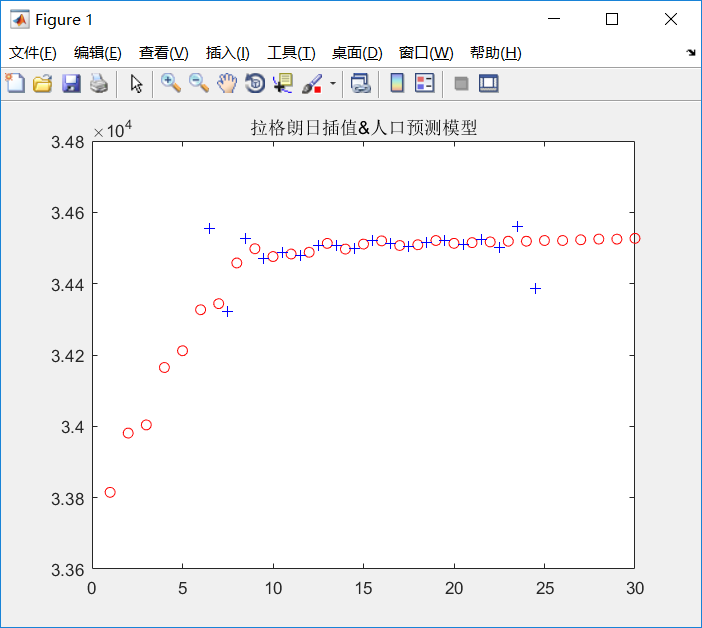人口数据给了30个已知数据点，属于是高次插值，可以看出效果并不理想
代码：
clear all
clc
% 人口数据
T=1:30;  % +1970=年份
P=[33815 33981 34004 34165 34212 34327 34344 34458 34498 34476 34483 34488 34513 34497 34511 34520 34507 34509 34521 34513 34515 34517 34519 34519 34521 34521 34523 34525 34525 34527];%人口
%plot(T,P)% 折线
plot(T,P,'r o')% 只画数据点
hold on
x1=1.5:30.5;
y1=Lagrange(T,P,x1);
plot(x1,y1,'b +')
axis([0 30 33600 34800])
title('拉格朗日插值&人口预测模型')

function y1=Lagrange(x,y,x1);
% x   插值节点
% y   被插值函数在节点x处的函数值
% x1  待插值节点
% y1  待插值节点的插值结果
n=length(x); % 数据点个数
m=length(x1); % 待插值点数
for k=1:m
z=x1(k);
sum=0.0;
for i=1:n
prod = 1.0;
for j=1:n
if j~=i
prod = prod * (z - x(j)) / (x(i) - x(j));
end
end
sum = sum + prod * y(i);
end
y1(k) = sum;
end

1.1.2 牛顿多项式插值法（差商）
插值的思想和目的还是一样的

牛顿插值法的公式是另一种n次插值多项式的构造形式，然而它却克服了拉格朗日插值多项式的缺陷，它的一个显著优势就是每当增加一个插值节点，只要在原牛顿插值公式中增加一项就可形成高一次的插值公式。此外，如果在实际应用中遇到等距分布的插值节点，牛顿插值公式就能得到进一步的简化，从而得到等距节点的插值公式，这样为缩短实际运算时间做出了很大的贡献。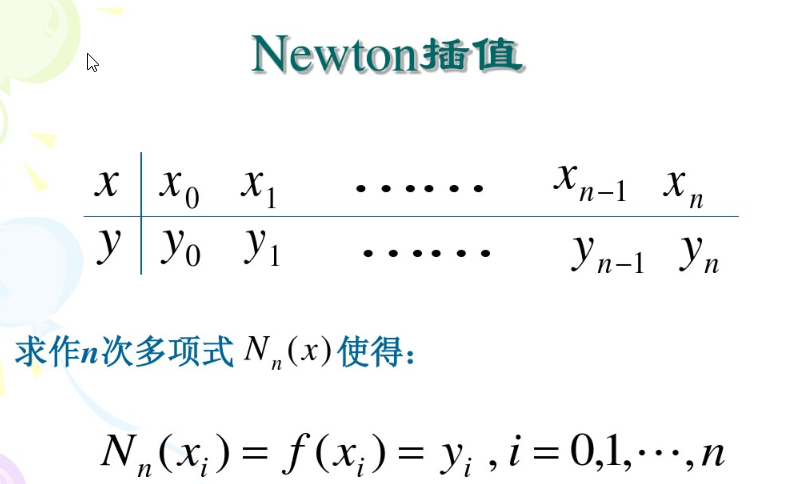差商
一个挺有意思的东西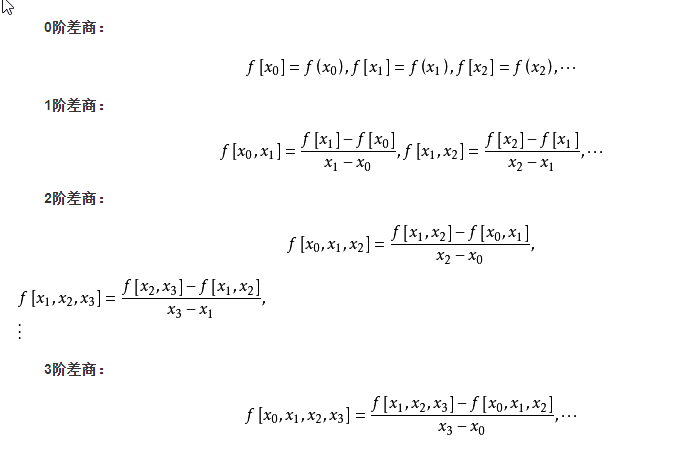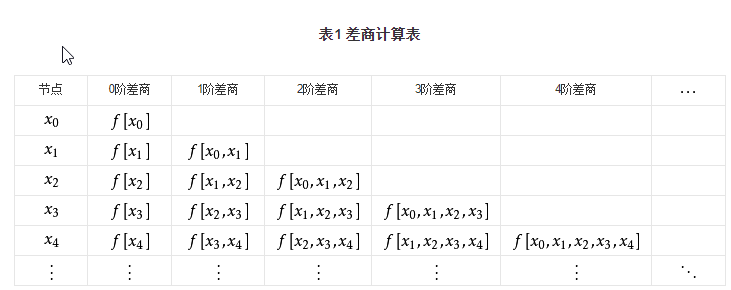##### 差商矩阵
理解差商矩阵是理解牛顿插值代码的灵魂之门
(以5个插值数据点为例，则差商矩阵为5*5)
matlab中索引从1开始，我就保持一致，不从0开始了

y(1)

y(2)
$\frac{y(2)-y(1)}{x(2)-x(1)}$, i.e. $f[x_1,x_2]$

y(3)
$\frac{y(3)-y(2)}{x(3)-x(2)}$, i.e. $f[x_2,x_3]$
$\frac{f[x_2,x_3]-f[x_1,x_2]}{x(3)-x(1)}，i.e. f[x_1,x_2,x_3]$

y(4)
$\frac{y(4)-y(3)}{x(4)-x(3)}$, i.e. $f[x_3,x_4]$
$f[x_2,x_3,x_4]$
$f[x_1,x_2,x_3,x_4]$

y(5)
$\frac{y(5)-y(4)}{x(5)-x(4)}$, i.e. $f[x_4,x_5]$
$f[x_3,x_4,x_5$]
$f[x_2,x_3,x_4,x_5]$
$f[x_1,x_2,x_3,x_4,x_5]$

计算关键代码：
 A(i,j) = (A(i,j-1) - A(i-1,j-1))/(X(i)-X(i-j+1));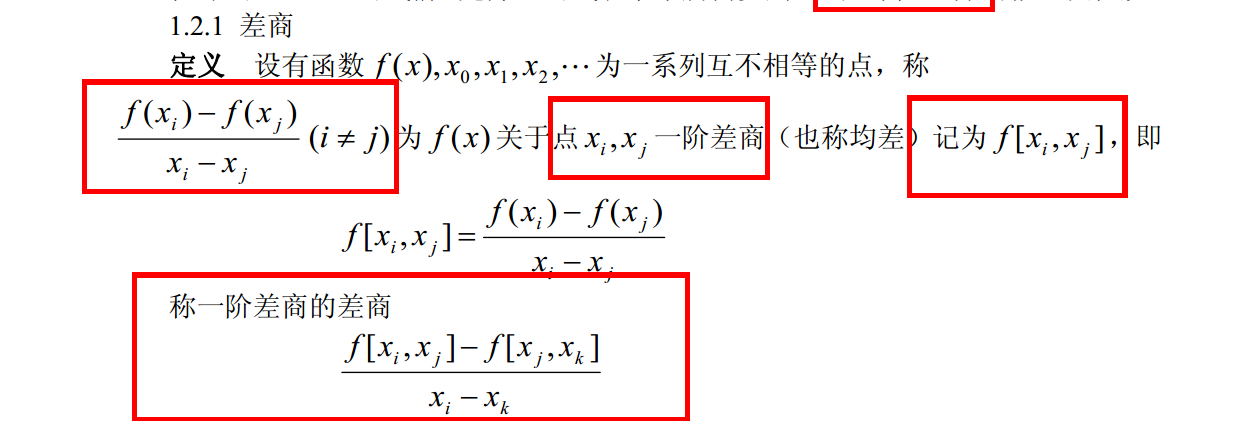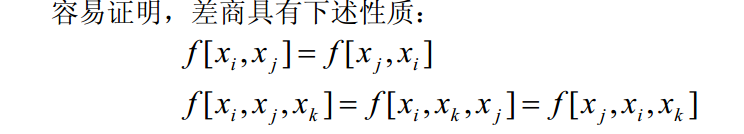示例
此代码被撸了仨小时，参考的一位博主的非常好的代码，把差商用一个矩阵存放，还计算了插值余项，链接
牛顿插值的理论可以在链接里看，讲的很详细
%newton.m
%求牛顿插值多项式、差商、插值及其误差估计的MATLAB主程序
%输入的量:X是n+1个节点(x_i,y_i)(i = 1,2, ... , n+1)横坐标向量，Y是纵坐标向量，
%x是以向量形式输入的m个插值点，M在[a,b]上满足｜f~(n+1)(x)｜≤M
%注：f~(n+1)(x)表示f(x)的n+1阶导数
%输出的量：向量y是向量x处的插值，误差限R，n次牛顿插值多项式L及其系数向量C，
%差商的矩阵A
function[y,R,A,C,L] = newton(X,Y,x,M)
n = length(X);
m = length(x);
for t = 1 : m
z = x(t);
A = zeros(n,n);
A(:,1) = Y';
s = 0.0; p = 1.0; q1 = 1.0; c1 = 1.0;
for j = 2 : n
for i = j : n
A(i,j) = (A(i,j-1) - A(i-1,j-1))/(X(i)-X(i-j+1));
end
q1 = abs(q1*(z-X(j-1))); % 余项计算中的差值连乘项
c1 = c1 * j; % 余项计算中的分母，阶乘
end
C = A(n, n); q1 = abs(q1*(z-X(n)));
for k = (n-1):-1:1
C = conv(C, poly(X(k)));
% poly函数把根转换为求得此根的多项式，X（k）是一个数，所以转换为一次多项式，得到其系数向量
% conv函数的输入是多项式的系数向量时，相当于直接相乘
d = length(C);
C(d) = C(d) + A(k,k);%在最后一维，也就是常数项加上新的差商
end
y(t) = polyval(C,z); % 插值结果
R(t) = M * q1 / c1; % 用M代替f~(n+1)(x)，即f(x)的n+1阶导数，这样求得的余项比真实值略大
end
L = vpa(poly2sym(C),3);% vpa让输出结果系数为小数而非分数,3表示用3位小数

测试脚本：
clear all
clc
X = [0 pi/6 pi/4 pi/3 pi/2];
Y = [0 0.5 0.7071 0.8660 1];
x = linspace(0,pi,50);
M = 1;
[y,R,A,C,L] = newton(X, Y, x, M);
y1 = sin(x);
errorbar(x,y,R,'.g')
hold on
plot(X, Y, 'or', x, y, '.k', x, y1, '-b');
legend('误差','样本点','牛顿插值估算','sin(x)');

这里已知数据点少，多项式次数低，插值效果就比较好
插值效果：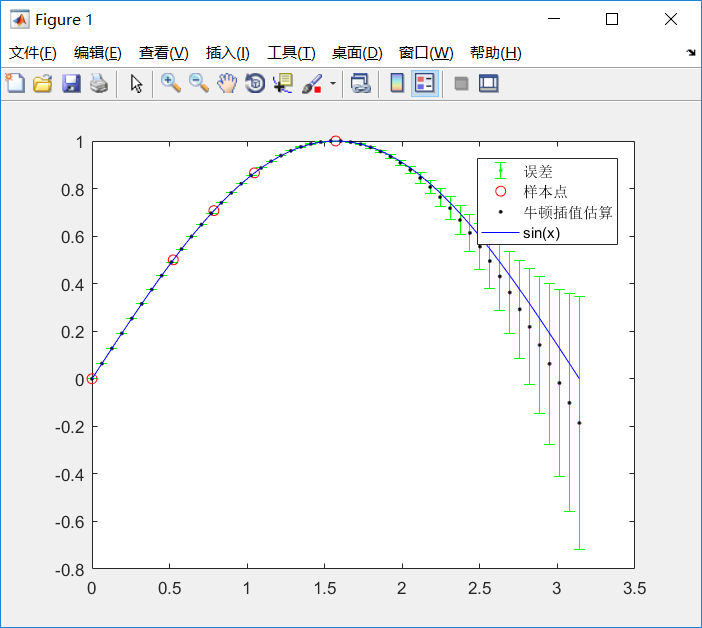计算出的牛顿插值多项式，多项式系数，差商矩阵：
>> C
C =
0.0290   -0.2048    0.0217    0.9955         0
>> L
L =
0.029*x^4 - 0.205*x^3 + 0.0217*x^2 + 0.996*x
>> A
A =
0         0         0         0         0
0.5000    0.9549         0         0         0
0.7071    0.7911   -0.2086         0         0
0.8660    0.6070   -0.3516   -0.1365         0
1.0000    0.2559   -0.4469   -0.0910    0.0290

另一套数据的插值结果：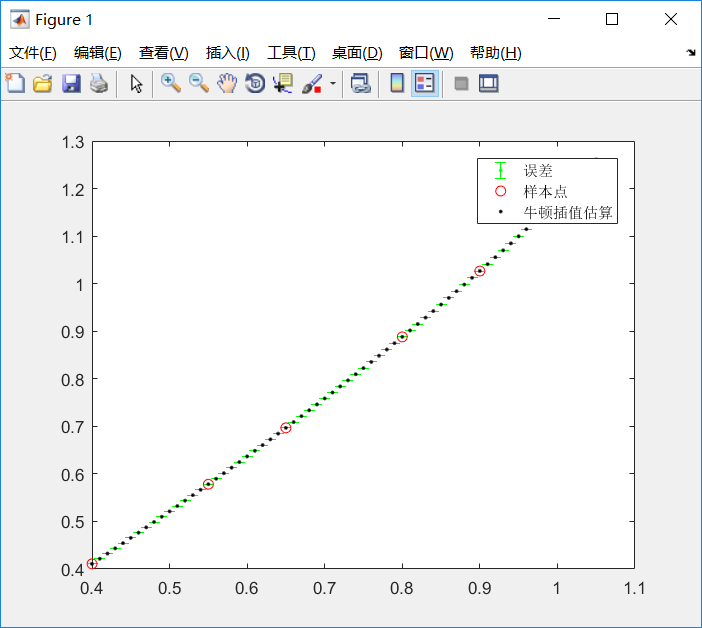代码：
X=[0.4 0.55 0.65 0.80 0.90 1.05];
Y=[0.41075 0.57815 0.69675 0.88811 1.02652 1.25386];
x=0.4:0.01:1.05;
M = 1;
[y,R,A,C,L] = newton(X, Y, x, M);
errorbar(x,y,R,'.g')
hold on
plot(X, Y, 'or', x, y, '.k');
legend('误差','样本点','牛顿插值估算');

用于人口数据插值：
惨不忍睹的结果······
所以并没有一种插值可以通用，完美拟合任何数据，要选择适合数据的插值方式
这个人口数据呈明显的对数函数趋势，要用对数型函数去拟合（见这篇博客）。而**不能插值！！！**除非用于预测，某年数据还说得过去
这里的数据点有30个，很多，牛顿插值得到了29次多项式, 共30个系数，高次多项式的函数图像很容易震荡，下图也可以看出插值数据的震荡性，这使得得到的插值函数并不光滑，而人口数据本身是很光滑的对数形状的曲线，这无法保证插值函数处处收敛于被插值函数（就是原数据点真正遵循的那个函数），这是高次多项式插值的通病。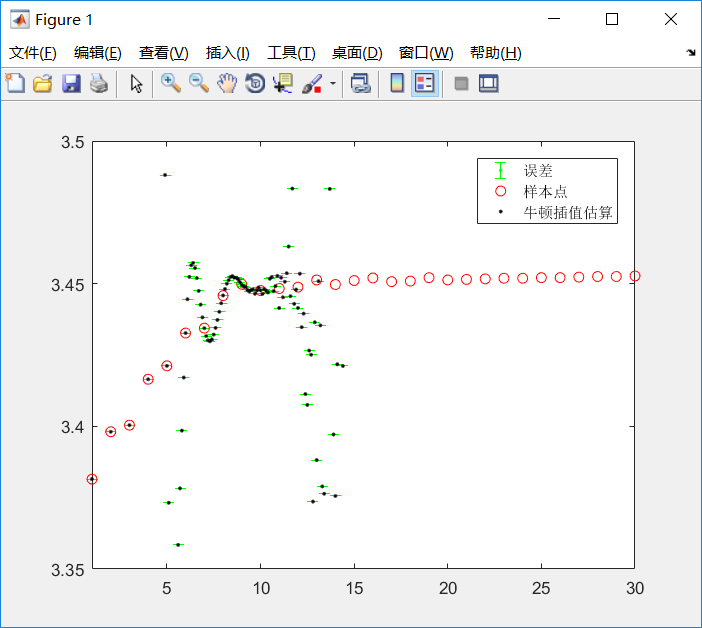代码：
clear all
clc
% 人口数据
T=1:30;  % +1970=年份
P=[33815 33981 34004 34165 34212 34327 34344 34458 34498 34476 34483 34488 34513 34497 34511 34520 34507 34509 34521 34513 34515 34517 34519 34519 34521 34521 34523 34525 34525 34527];%人口
P=P/10000;
M = 1;
x=1:.1:30;
[y,R,A,C,L] = newton(T, P, x, M);
errorbar(x,y,R,'.g')
hold on
axis([1 30 3.35 3.50])
plot(T, P, 'or', x, y, '.k');
legend('误差','样本点','牛顿插值估算');

低次多项式插值（不易震荡）的其他示例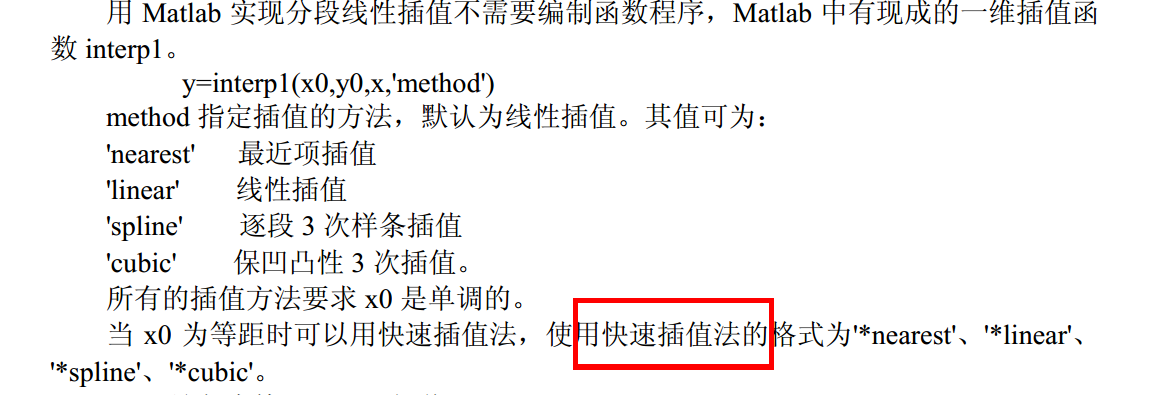保凹凸性即hermite插值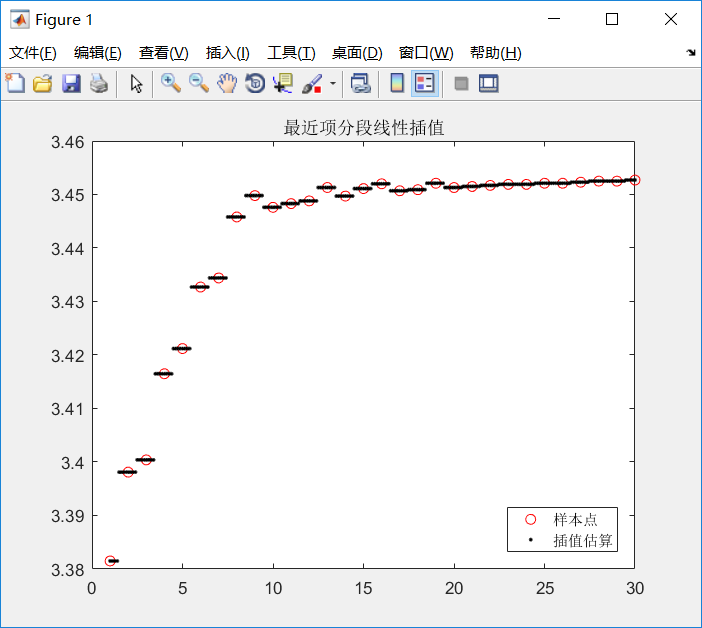最差无疑了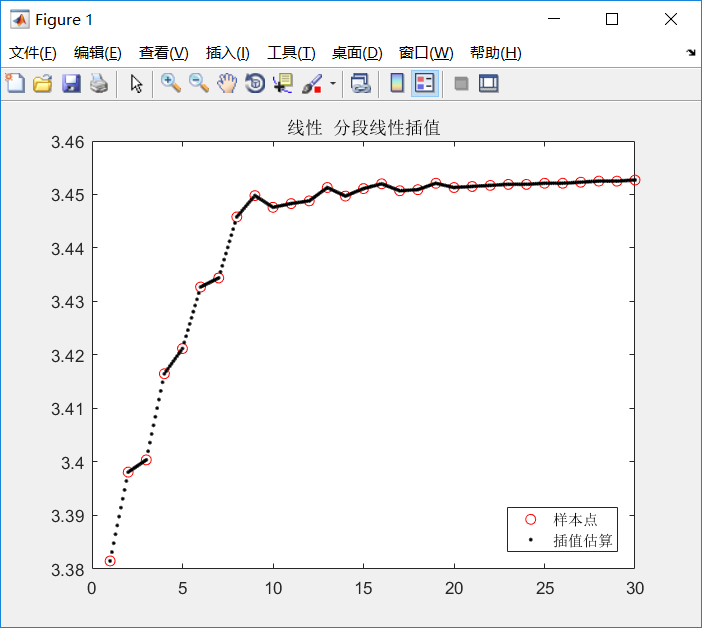分段线性插值的子插值函数都是一次的，从图像上也可以看得出来，一段一段的，很不光滑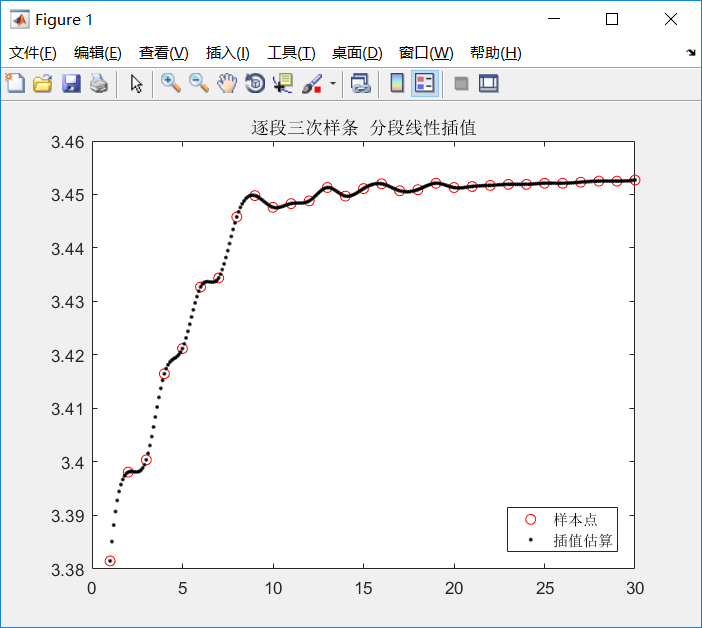三次样条的结果明显要光滑很多，子插值函数都是三次的，还考虑了一二阶导数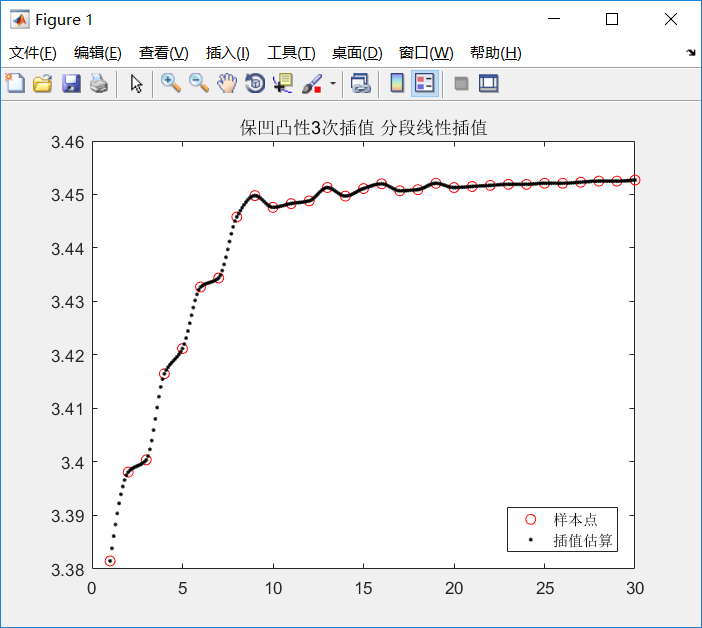代码：
clear all
clc
% 人口数据
T=1:30;  % +1970=年份
P=[33815 33981 34004 34165 34212 34327 34344 34458 34498 34476 34483 34488 34513 34497 34511 34520 34507 34509 34521 34513 34515 34517 34519 34519 34521 34521 34523 34525 34525 34527];%人口
P=P/10000;
x=1:.1:30;
%y=interp1(T,P,x,'*nearest') ;
%y=interp1(T,P,x,'*linear') ;
%y=interp1(T,P,x,'*spline') ;
y=interp1(T,P,x,'*cubic') ;
axis([1 30 3.35 3.50])
plot(T, P, 'or', x, y, '.k');
legend('样本点','插值估算','Location','southeast');
%title('最近项分段线性插值')
%title('线性  分段线性插值')
% title('逐段三次样条  分段线性插值')
title('保凹凸性3次插值 分段线性插值')

% 'north' 坐标轴中的顶部
% 'south' 坐标轴中的底部
% 'east' 坐标轴中的右侧区域
% 'west' 坐标轴中的左侧区域
% 'northeast' 坐标轴中的右上角（二维坐标轴的默认值）
% 'northwest' 坐标轴中的左上角
% 'southeast' 坐标轴中的右下角
% 'southwest' 坐标轴中的左下角
% 'northoutside' 坐标轴的上方
% 'southoutside' 坐标轴的下方
% 'eastoutside' 到坐标轴的右侧
% 'westoutside' 到坐标轴的左侧
% 'northeastoutside' 坐标轴外的右上角（三维坐标轴的默认值）
% 'northwestoutside' 坐标轴外的左上角
% 'southeastoutside' 坐标轴外的右下角
% 'southwestoutside' 坐标轴外的左下角
% 'best' 坐标轴内与绘图数据冲突最少的地方
% 'bestoutside' 到坐标轴的右侧
% 'none' 由 Position 属性决定。可使用 Position 属性在自定义位置显示图例。

尝试用在样本数据点少的情况：
对sin函数插值（前面用牛顿插值取得了不错的效果），可以看到和真实sin函数在后面差距越来越大。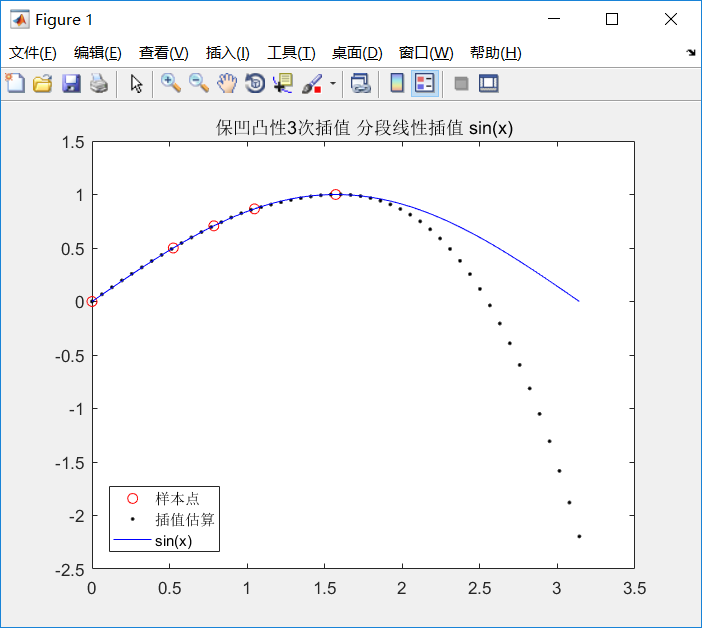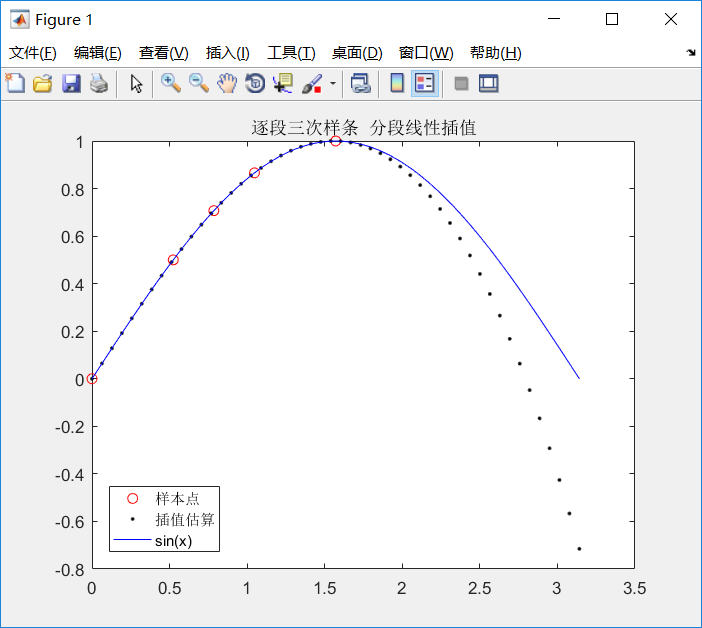这时候最近项线性插值是完全不能用的：
可以看出最近项分段线性插值只能用于数据点非常非常多的情况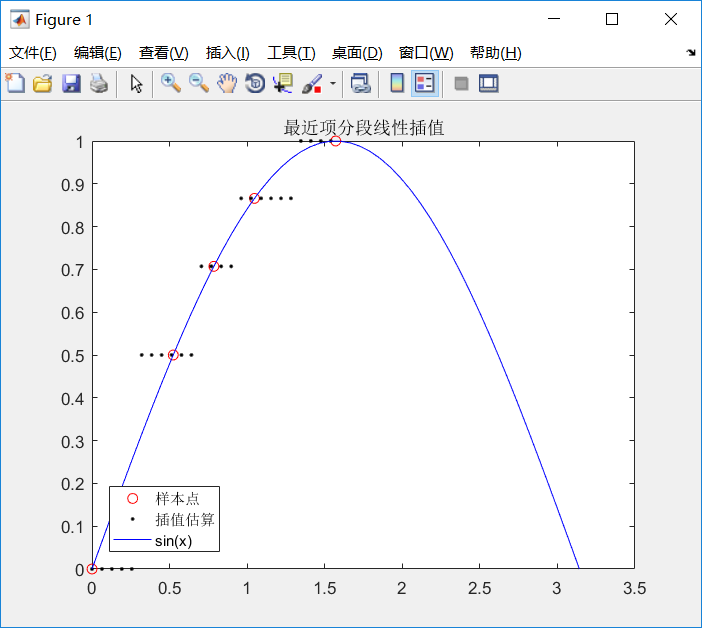linear分段线性插值也不适合数据点少：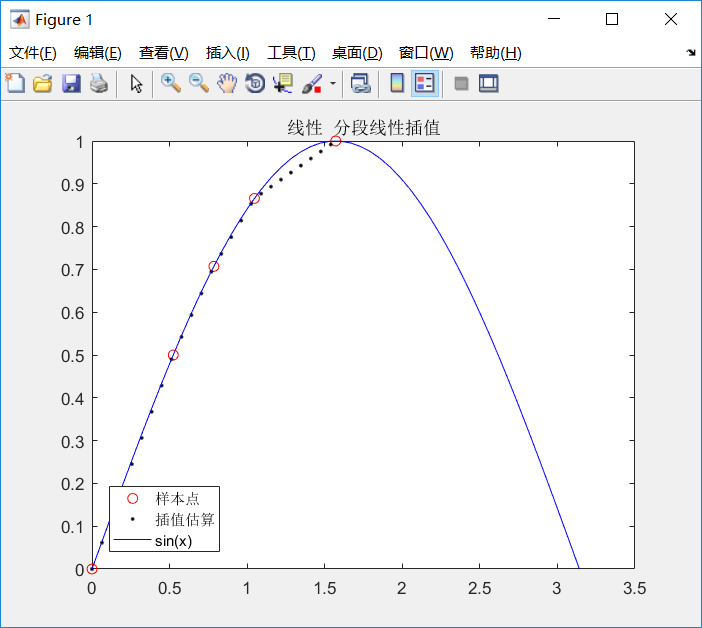1.1.3 拉格朗日插值和牛顿插值的对比
不同
前者不具有继承性导致计算量大
后者有继承性，数据点增多只需要在原来的多项式基础上增加几项，计算量要小一些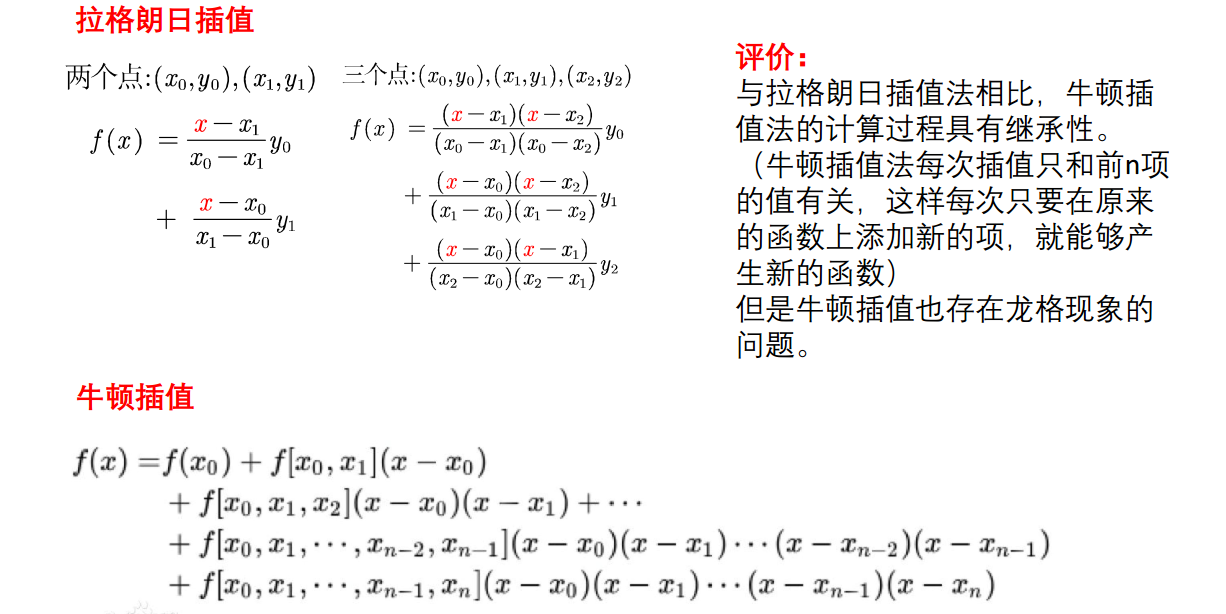相同
都存在龙格效应
都没有考虑导数也要相同，所以可能不能模拟出被插值函数的形态，所以就引入了后面的考虑导数的插值法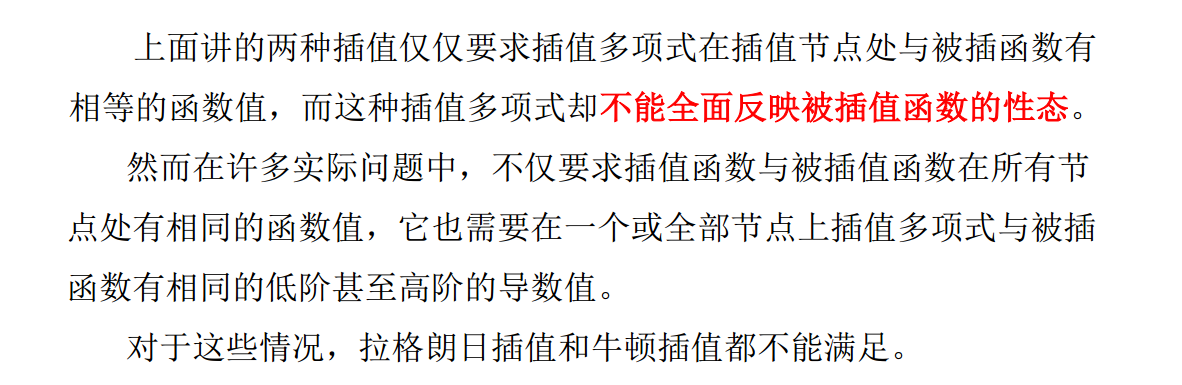1.2 考虑导数的插值方法
1.2.1Hermite插值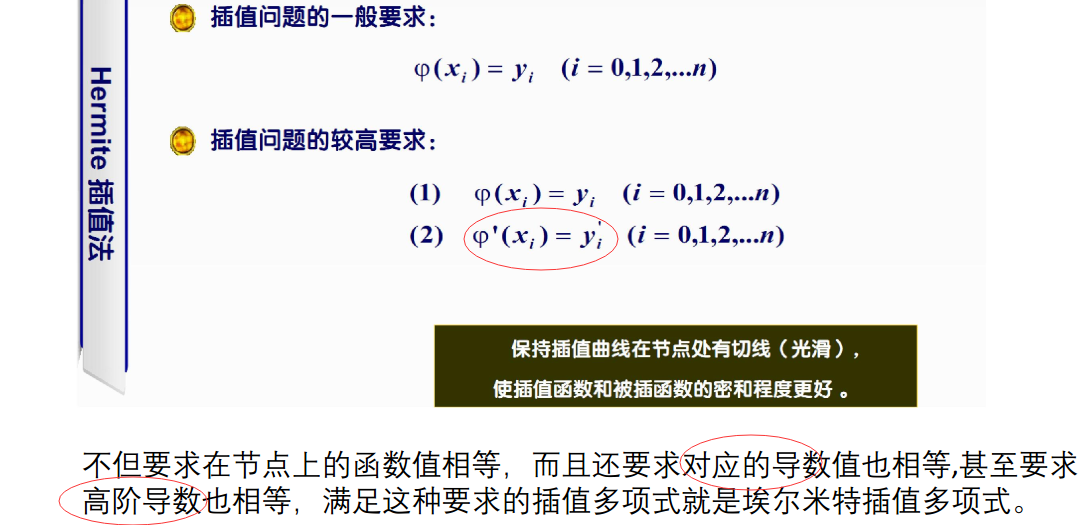分段三次hermite插值
有前途，就用这个了，比分段线性插值好呀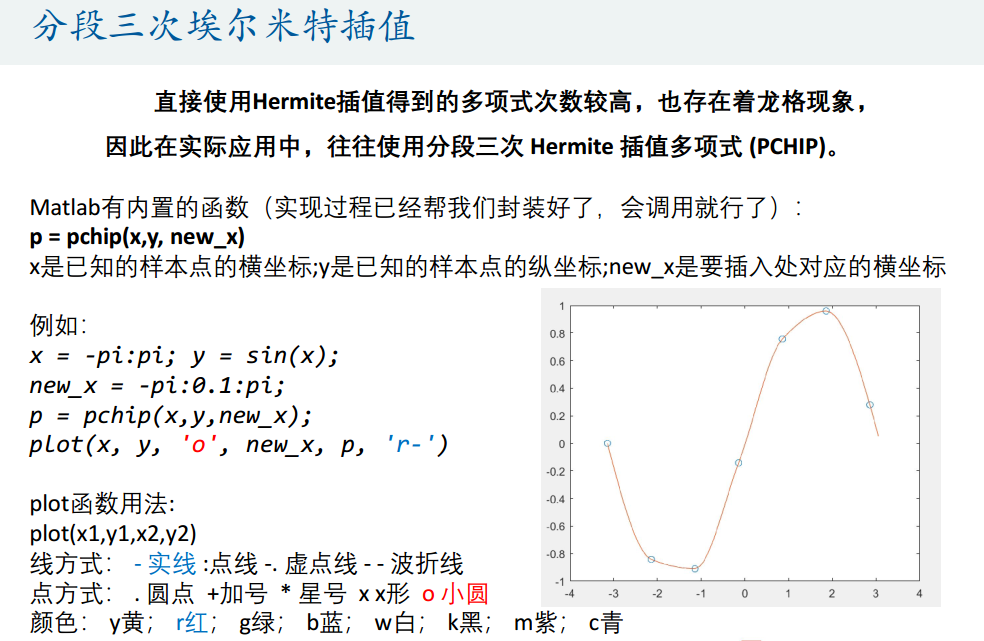优点：关于插值函数和被插函数的贴合程度，埃尔米特插值比多项式的好。
缺点：埃尔米特插值只有在被插值函数在插值节点处的函数值和导数值已知时才可以使用，而这在实际问题中是无法实现的，因为在一般情况下我们是不可能也没必要知道函数在插值节点处的导数值。因此成为能否运用埃尔米特插值的一个重要因素就是：我们知不知道插值函数在节点处的导数值。

1.2.2 三次样条插值
定义
每个子区间的多项式函数是三次的，所以名字里有“三次”，就像前面拉格朗日插值的分段二次插值的每个子区间的插值函数是二次的一样
整个插值函数曲线由分段三次曲线并接而成，并且在连接点也就是样点上必须要二阶连续可导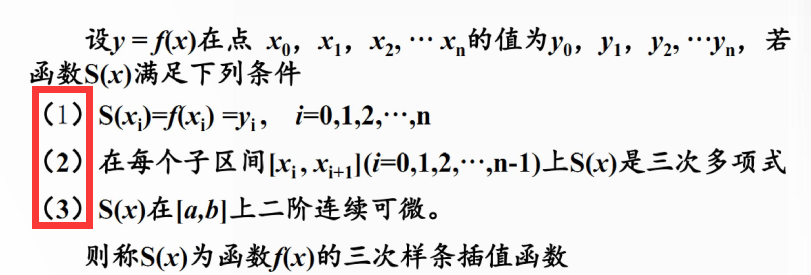n+1个数据点，共有n个分段多项式
相邻两个子多项式之间必须在函数值，一阶导数，二阶导数上都相等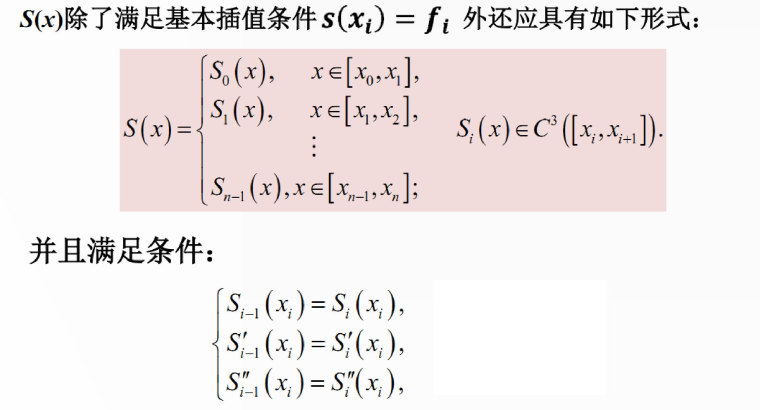对比发现，分段三次hermite插值只考虑了一阶导数，分段三次样条考虑了一阶导数和二阶导数，所以后者得到的结果更加精确
示例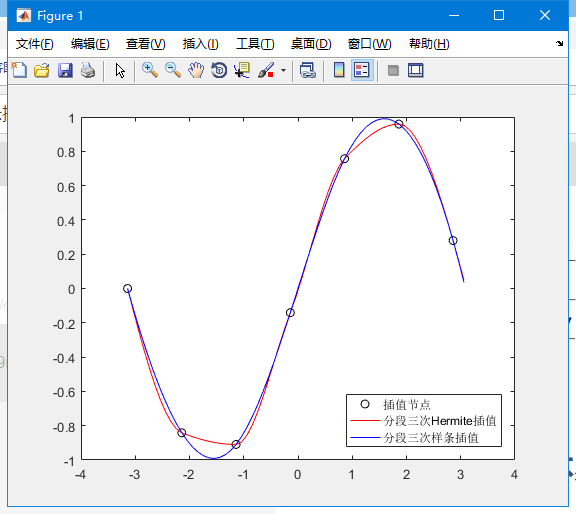代码
x = -pi:pi;
y = sin(x);
x_ = -pi:.1:pi;
p1 = pchip(x,y,x_); % 分段三次Hermite插值
p2 = spline(x,y,x_); % 分段三次样条插值
plot(x,y,'ko',x_,p1,'r-',x_,p2,'b-')
legend('插值节点','分段三次Hermite插值','分段三次样条插值','location','southeast')

可见，三次样条的结果更加光滑，也更接近sin函数本身的图像
用插值进行数据预测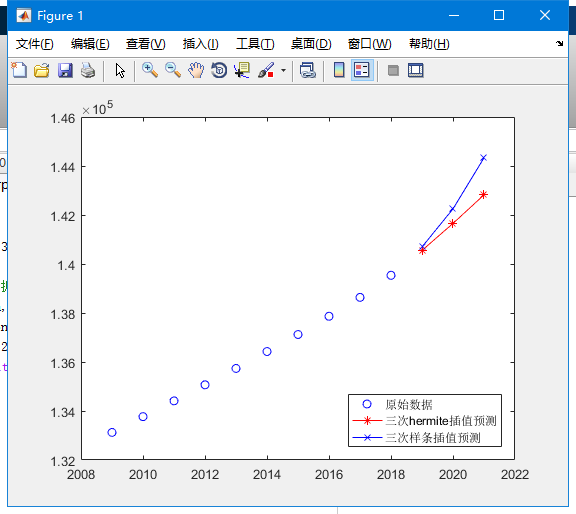代码
% 用插值进行预测
% 2009-2018 10年的人口数据
population=[133126,133770,134413,135069,135738,136427,137122,137866,138639, 139538];
year = 2009:2018;
% 预测 2019-2021三年的人口数据
p1 = pchip(year, population, 2019:2021);
p2 = spline(year, population, 2019:2021);
plot(year, population,'bo',2019:2021,p1,'r*-',2019:2021,p2,'bx-')
legend('原始数据','三次hermite插值预测','三次样条插值预测','location','southeast')

总结(必看+1)

拉格朗日插值、牛顿插值、分段线性插值、分段三次Hermite插值和分段三次样条插值具有不同的优势和适用范围，对于方法的选择至关重要，我们需要对它们进行差异化的了解与认知。

分段插值函数比如分段线性插值、3次分段hermite和三次分段样条插值函数在每个单元区间上收敛性强，数值稳定性好且易于计算机编程实现

一般来说，不要直接使用多项式插值，尤其是数据点较多（n>7）时，高次多项式震荡很厉害；
分段多项式插值可以避免震荡带来的误差；
分段多项式插值又不如分段hermite，因为后者考虑了一阶导数；
分段hermite又不如分段三次样条，因为后者除了一阶导数，还考虑了二阶导数，更好地保持数据的光滑性和连续性，减少信息量的损失。

回顾上面的所有示例图，一般来说，使用逐段三次样条可以获得最优插值结果，由于我们不知道数据本身的分布，所以分段三次hermite也可以尝试。其他的当然也是可以使用的，根据自己的问题自己判断吧

一般来说，使用逐段三次样条可以获得最优插值结果
一般来说，使用逐段三次样条可以获得最优插值结果
一般来说，使用逐段三次样条可以获得最优插值结果
还是要分情况的，最近发现，数据量很大时，比如一条数据向量三千多维，给一千个索引，得到的插值结果在两端会出现强烈的龙格现象，很大的甩尾swings，也是一个棘手的山芋
如果给的索引不是从1开始，linear 和nearest竟然不给两端插值！！！！！我也是服气，整出NAN玩儿
K>> interp1([3 5 7],[4 3 6],1:9,'linear')

ans =

NaN       NaN    4.0000    3.5000    3.0000    4.5000    6.0000       NaN       NaN

K>> interp1([3 5 7],[4 3 6],1:9,'nearest')

ans =

NaN   NaN     4     3     3     6     6   NaN   NaN

K>> interp1([3 5 7],[4 3 6],1:9,'cubic')
警告: 在以后的版本中将会更改 INTERP1(...,'CUBIC')。请改用 INTERP1(...,'PCHIP')。
> In interp1>sanitycheckmethod (line 265)
In interp1>parseinputs (line 401)
In interp1 (line 80)
In feature_extract (line 393)

ans =

11.0000    6.3750    4.0000    3.1250    3.0000    3.8750    6.0000    8.6250   11.0000

K>> interp1([3 5 7],[4 3 6],1:9,'spline')

ans =

9     6     4     3     3     4     6     9    13



展开全文matlab
• 本篇主要介绍在三种插值方法：拉格朗日插值、分段线性插值、三次样条插值，以及这三种方法在matlab中如何实现。 1.拉格朗日插值：  1.1基本原理：先构造组基函数：   是次多项式，满足 令 上式称为...
本篇主要介绍在三种插值方法：拉格朗日插值、分段线性插值、三次样条插值，以及这三种方法在matlab中如何实现。

1.拉格朗日插值：

1.1基本原理：先构造一组基函数：

是次多项式，满足

令

上式称为次Lagrange插值多项式。

1.2用Matlab作Lagrange插值：

matlab没有现成的lagrange函数，需要手动写，如下：

x0，y0为原始坐标点，维度必须相同。

x为待插值的点。

y是返回值，是最终插值结果。

function y=lagrange(x0,y0,x)   %x0,y0为初始坐标，x为需要插值的点，返回的y为插值结果
n=length(x0);m=length(x);
for i=1:m
z=x(i);
s=0;
for j=1:n
p=1;
for k=1:n
if k~=j
p=p*((z-x0(j))/(x0(k)-x0(j)));
end
end
s=p*y0(k)+s;
end
y(i)=s;
end

2.分段线性插值：

2.1基本原理：

将每两个相邻的节点用直线连起来，如此形成的一条折线就是分段线性插值函数。计算点的插值时，只用到左右的两个节点，计算量与节点个数n（初始值x0，y0的长度,n=length(x0)）无关，而拉格朗日插值与n值有关。分段线性插值中n越大，分段越多，插值误差越小。

2.2Matlab实现分段线性插值：

用matlab实现分段线性插值不需要自己手动编写函数，matlab有现成的一维插值函数interp1

y=interp1(x0,y0,x,'method')

method指定插值方法，其值可为：

linear：线性插值（默认）

nearest：最近项插值

spline：逐次3次样条插值

cubic：保凹凸性 3 次插值

所有插值方法都要求x0单调。

3.三次样条插值：

使用三次样条插值有两种方法：其中一种就是第二种插值方式（分段线性插值），只需要将method修改为spline即可实现。

还有一种实现方式如下：

pp=csape(x0,y0);

y=ppval(pp,x);

其中x0，y0，x与前面含义相同，返回值y即插值结果。

4.彩蛋（例题）：

表1给出的 x, y 数据位于机翼断面的下轮廓线上，假设需要得到 x 坐标每改变0.1 时的 y 坐标。试完成加工所需数据，画出曲线。要求用 Lagrange、分段线性和三次样条三种插值方法计算。

表1

x   0 3 5 7 9 11 12 13 14 15
y   0 1.2 1.7 2.0 2.1 2.0 1.8 1.2 1.0 1.6
解：编写代码如下：

clear,clc
x0=[0,3,5,7,9,11,12,13,14,15];
y0=[0,1.2,1.7,2.0,2.1,2.0,1.8,1.2,1.0,1.6];
x1=[0:0.1:15];

%拉格朗日插值
y1=lagrange(x0,y0,x1);
figure
plot(x0,y0,x1,y1,'.')
title('拉格朗日插值')

%分段线性插值
y2=interp1(x0,y0,x1);
figure
plot(x0,y0,x1,y2,'.')
title('分段线性插值')

%三次样条插值
y3=interp1(x0,y0,x1,'spline');
figure
plot(x0,y0,x1,y3,'.')
title('三次样条插值')



结果图为: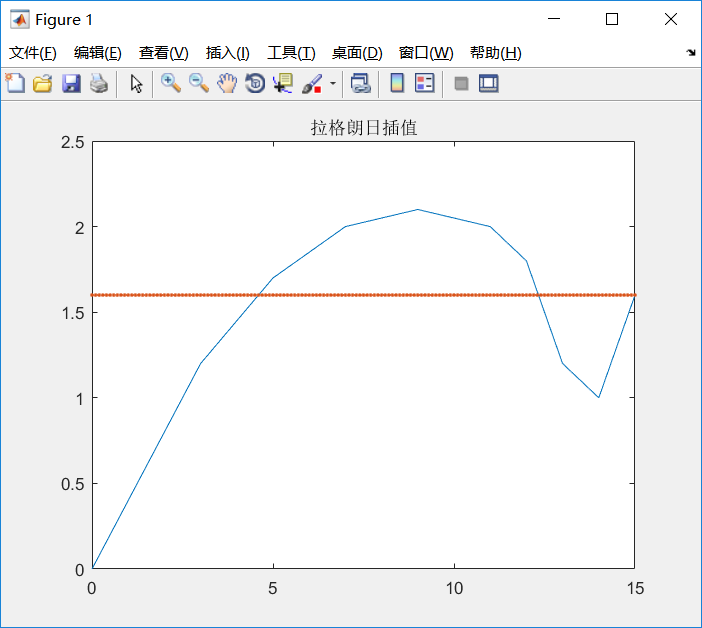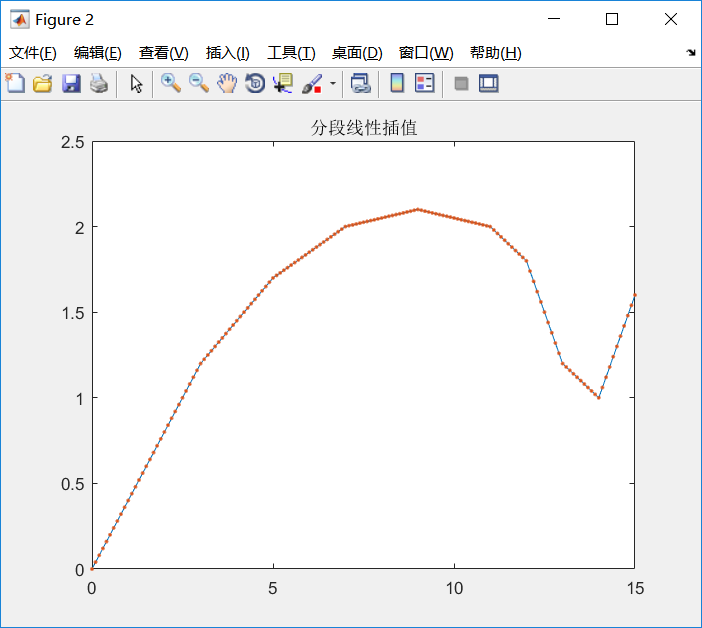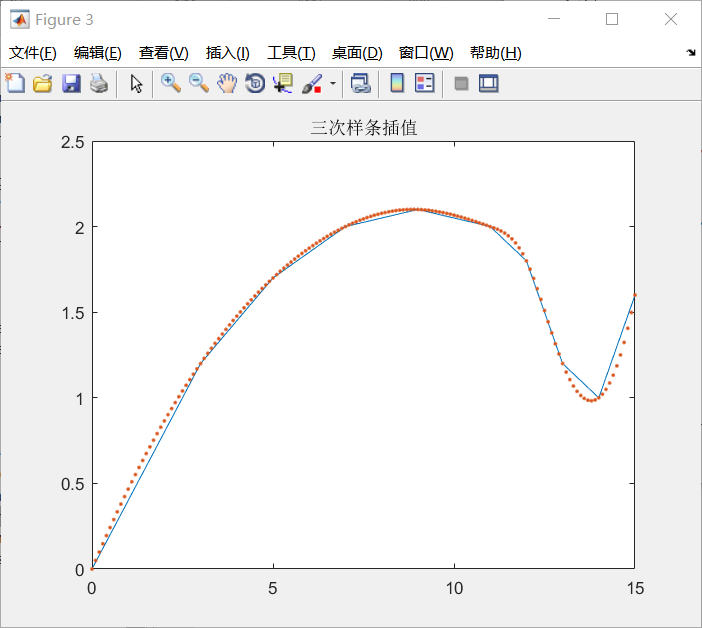由图可知：拉格朗日插值误差较大，插值大量点的时候不建议使用。分段线性插值结果最好，使用的是method是linear（默认）。三次样条插值有点误差，但不是很大，得到的曲线比较光滑。

学习插值函数时的一点小心得，希望能帮到大家！
展开全文matlab
• 主要介绍了Numpy一维线性插值函数的用法，具有很好的参考价值，希望对大家有所帮助。一起跟随小编过来看看吧
• reference:https://blog.csdn.net/u013355826/article/details/56680521 简单的理解过程就在上面的bolg链接，非常有用，从不理解插值到会使用
reference:https://blog.csdn.net/u013355826/article/details/56680521
简单的理解过程就在上面的bolg链接，非常有用，从不理解插值到会使用


展开全文• x0=[0 3 5 7 9 11 12 13 14 15 ]; y0=[0 1.2 1.7 2.0 2.1 2.0 1.8 1.2 1.0 1.6 ]; x=0:0.1:15; y1=interp1(x0,y0,x); y2=interp1(x0,y0,x,'spline'); subplot(2,1,1) plot(x0,y0,'k+',x,y1,'r') ...
x0=[0 3 5 7 9 11 12 13 14 15 ];
y0=[0 1.2 1.7 2.0 2.1 2.0 1.8 1.2 1.0 1.6 ];
x=0:0.1:15;
y1=interp1(x0,y0,x);
y2=interp1(x0,y0,x,'spline');
subplot(2,1,1)
plot(x0,y0,'k+',x,y1,'r')
grid
title('piecewise linear')
subplot(2,1,2)
plot(x0,y0,'k+',x,y2,'r')
grid
title('spline')



展开全文matlab
• 在用生成对抗网络生成二维数据点的时候遇到代码里的一个问题，就是numpy中的一维线性插值函数interp到底是怎么用的，在这个上面费了点功夫，因此现将其用法给出。 在生成对抗网络的二维样本生成的例子中，涉及了...
• 插值：求过已知有限个数据点的近似函数。 拟合：已知有限个数据点，求近似函数，不要求过已知数据点，只要求在某种意义下它在这些点...下面介绍几种基本的、常用的插值：拉格朗日多项式插值、牛顿插值分段线性插...
• 三线性插值（trilinear interpolation）主要是用于在个3D的立方体中，通过给定顶点的数值然后计算立方体中其他点的数值的线性插值方法。 具体推导过程见参考资料1，这里直接给出最终公式： 其中，坐标(x,y,z...线性插值 三线性插值
• x - 表示将要计算的插值点x坐标 xp - 表示已有的xp数组 fp - 表示对应于已有的xp数组的值 left - 表示当x值在xp中最小值左边时，x对应y的值为left right -表示当x值在xp中最大值右边时，x对应y的值为ri...
• ## Liner(分段线性插值)

万次阅读 2015-09-08 17:01:07算法
• interpl( )一维插值函数 格式： yi＝interpl (x, y, xi, 'method') 功能：为给定的数据对(x,y)以及x坐标上的插值范围向量xi，用指定所使用的插值方法method实现插值。yi是插值后的对应数据点集的y坐标 简单来...interp1 interp2 matlab 插值函数
• ## 分段线性插值 PWL

千次阅读 2019-10-07 20:42:21
分段线性插值在速度误差取得了很好的平衡，插值函数具有连续性，但是由于在已知点的斜率是不变的，所以插值结果不光滑。 第次用matlab写代码，有点手生。 liner.m文件： function yy = liner(x,y,xx) %x是已...
• 本文给出个用C语言实现全程线性分段插值算法的程序。
• ++仅限标头库，用于对非结构化数据进行N维分段线性插值，类似于MatlabSciPy的命令。 假设我们得到了组数据点(x, f(x)) ，其中x是N的。 这个想法是构造数据点的x坐标的N。 三角剖分中的每个顶点对应个数据...
• 1、面向一维插值线性拟合，含有牛顿插值、线性插值等四种插值方式； 2、设计了可用鼠标直接操作的GUI界面
• 问题 已知 XY有下表的对应关系，当个新的4<...采用线性插值的方法，找到x前后两个数x1x2，线性计算 y=y1 + x*(y2-y1)/(x2-x1)即可 因此 x=10，y应该是85 Excel函数介绍 trend（）函数 说明 返...excel 算法
• 将TXT文件中的数据读进二数组，并对列数据进行线性插值再以txt文件将数据输出
• ## 分段线性插值

千次阅读 2005-06-12 16:16:00
/*****分段线性插值*** 计算公式：S1(x)=Φ0（x-x(i)/h(i))）y(i)+Φ1（x-x(i)/h(i)）y(i+1) 步长：h(i)=x(i+1)-x(i) 领域：x(i)≤x≤x(i+1) 属性：插值计算法 精度：| f(x) - S1(x) | = h(i)*h(i)/8 * max |...算法
• 在理解一维线性插值的基础上，我们可以去构建更高维度的插值算法。原理假设我们已知坐标(x0,y0)(x_0,y_0)与(x1,y1)(x_1,y_1)，要得到[x0,x1][x_0,x_1]区间内某一位置 xx 在直线上的值。根据图中所示，我们得到 y...c语言
• 特征点直接法 的 稀疏直接法 视觉里程计 最小化光度误差 灰度二维线性插值 * 空间点 P = (X, Y, Z) = (X, Y, Z, 1) 非齐次，齐次表示 (RGB-D获取3D坐标) * 两帧图像上 对应 的像素坐标 ...光度误差 稀疏直接法
• 目录 1.关于插值： ...线性插值是针对一维数据的插值方法。它根据一维数据序列中需要插值的点的左右临近两个数据来进行数值估计。当然了它不是求这两个点数据大小的平均值（在中心点的时候就等于平均...
• 在FPGA平台下，做乘除法需要专门的乘法器除法器。线性滤波器中，滤波器系数乘以信号，存在乘法。本文以HM中的TComPrediction::xPredIntraPlanar函数为例，介绍如何实现没有乘除法的线性滤波器。 TComPrediction::......